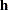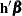The PHREG Procedure
 HAZARDRATIO Statement

HAZARDRATIO <’label’> variable </ options> ;

The HAZARDRATIO statement enables you to request hazard ratios for any variable in the model at customized settings. For example, if the model contains the interaction of a CLASS variable A and a continuous variable X, the following specification displays a table of hazard ratios comparing the hazards of each pair of levels of A at X=3:

```    hazardratio A / at (X=3) diff=ALL;
```

The HAZARDRATIO statement identifies the variable whose hazard ratios are to be evaluated. If the variable is a continuous variable, the hazard ratio compares the hazards for a given change (by default, a increase of 1 unit) in the variable. For a CLASS variable, a hazard ratio compares the hazards of two levels of the variable. More than one HAZARDRATIO statement can be specified, and an optional label (specified as a quoted string) helps identify the output.

Options for the HAZARDRATIO statement are as follows.

ALPHA=number

specifies the alpha level of the interval estimates for the hazard ratios. The value must be between 0 and 1. The default is the value of the ALPHA= option in the PROC PHREG statement, or 0.05 if that option is not specified.

AT (variable=ALL | REF | list <...variable=ALL | REF | list> )

specifies the variables that interact with the variable of interest and the corresponding values of the interacting variables. If the interacting variable is continuous and a numeric list is specified after the equal sign, hazard ratios are computed for each value in the list. If the interacting variable is a CLASS variable, you can specify, after the equal sign, a list of quoted strings corresponding to various levels of the CLASS variable, or you can specify the keyword ALL or REF. Hazard ratios are computed at each value of the list if the list is specified, or at each level of the interacting variable if ALL is specified, or at the reference level of the interacting variable if REF is specified.

If this option is not specified, PROC PHREG finds all the variables that interact with the variable of interest. If an interacting variable is a CLASS variable, variable= ALL is the default; if the interacting variable is continuous, variable=is the default, whereis the average of all the sampled values of the continuous variable.

Suppose the model contains two interactions: an interaction A*B of CLASS variables A and B, and another interaction A*X of A with a continuous variable X. If 3.5 is the average of the sampled values of X, the following two HAZARDRATIO statements are equivalent:

```hazardratio A;
hazardratio A / at (B=ALL X=3.5);
```
CL=WALD | PL | BOTH

specifies whether to create the Wald or profile-likelihood confidence limits, or both for the classical analyis. By default, Wald confidence limits are produced. This option is not applicable to a Bayesian analysis.

DIFF=ALL | REF

specifies which differences to consider for the level comparisons of a CLASS variable. The default is DIFF=ALL. This option is ignored in the estimation of hazard ratios for a continuous variable. DIFF=ALL requests all differences, and DIFF=REF requests comparisons between the reference level and all other levels of the CLASS variable.

E

displays the vectorof linear coefficients such thatis the log-hazard ratio, withbeing the vector of regression coefficients.

PLCONV=value

controls the convergence criterion for the profile-likelihood confidence limits. The quantity value must be a positive number, with a default value of 1E–4. The PLCONV= option has no effect if profile-likelihood confidence intervals (CL=PL) are not requested.

PLMAXIT=n

specifies the maximum number of iterations to achieve the convergence of the profile-likelihood confidence limits. By default, PLMAXITER=25. If convergence is not attained in n iterations, the corresponding profile-likelihood confidence limit for the hazard ratio is set to missing. The PLMAXITER= option has no effect if profile-likelihood confidence intervals (CL=PL) are not requested.

PLSINGULAR=value

specifies the tolerance for testing the singularity of the Hessian matrix in the computation of the profile-likelihood confidence limits. The test requires that a pivot for sweeping this matrix be at least this number times a norm of the matrix. Values of the PLSINGULAR= option must be numeric. By default, value is the machine epsilon times 1E7, which is approximately 1E–9. The PLSINGULAR= option has no effect if profile-likelihood confidence intervals (CL=PL) are not requested.

UNITS=value

specifies the units of change in the continuous explanatory variable for which the customized hazard ratio is estimated. The default is UNITS=1. This option is ignored in the computation of the hazard ratios for a CLASS variable.Previous Page | Next Page | Top of Page